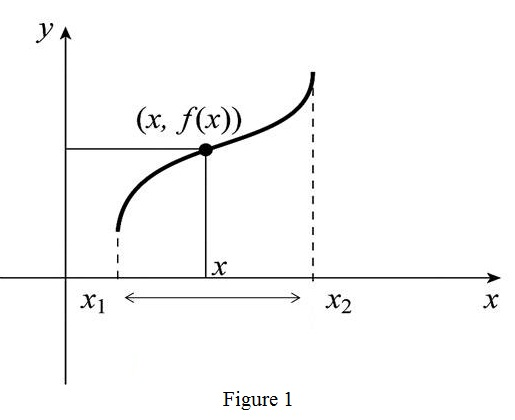Chapter 1, Problem 1RCC### Single Variable Calculus: Early Tr...

8th Edition
James Stewart
ISBN: 9781305270343

#### Solutions

Chapter
Section### Single Variable Calculus: Early Tr...

8th Edition
James Stewart
ISBN: 9781305270343
Textbook Problem

# (a) What is a function? What are its domain and range?(b) What is the graph of a function?(c) How can you tell whether a given curve is the graph of a function?

(a)

To determine

To explain: The terms function, domain of a function and range of a function.

Explanation

A function f is defined as a ordered pair (x,f(x)) such that x and f(x) are related by a definite rule.

The set of all such values of x is domain D for the function f and the set of all values of f(x) is called as range R.

(b)

To determine

To explain: The meaning of graph of the function f.

Explanation

Graph:

Draw the graph of the function f as shown in the Figure1As per the definition of the function it is an ordered pair (x,f(x)).

Plot the point (x,f(x))=(x,y) in the xy-plane for all values of x in the domain.

Connect all the points to obtain a curve this curve is known as graph of the function f.

(c)

To determine

To check: Whether the graph of a function exists or not.

Explanation

If the graph has no vertical line intersects to the curve more than once, then the graph of a function exists.

If the graph has the vertical line intersect to the curve more than once, then the graph of a function does not exist

#### The Solution to Your Study Problems

Bartleby provides explanations to thousands of textbook problems written by our experts, many with advanced degrees!

Get Started

## Additional Math Solutions

#### Find the median for the following set of scores: 1, 9, 3, 6, 4, 3, 11, 10

Essentials of Statistics for The Behavioral Sciences (MindTap Course List)

#### In Problem 31-38, write the equation of each line described. 38. Shown in the graph

Mathematical Applications for the Management, Life, and Social Sciences

#### Fill in the blanks. Applying De Morgans Laws, we can write (ABC)c=.

Finite Mathematics for the Managerial, Life, and Social Sciences

Find all the answers to your study problems with bartleby.
Textbook solutions plus Q&A. Get As ASAP arrow_forward